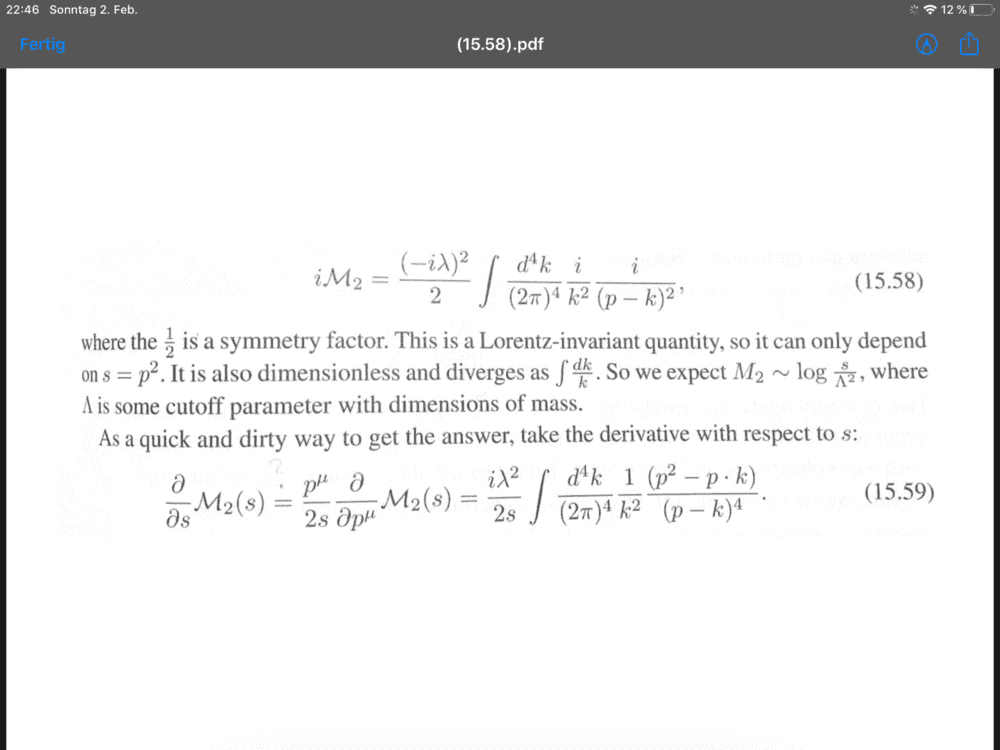# Schwartz QFT book, equation 15.59

• I
Pnin
Can someone please explain what the author does here in 15.59? I do not understand both steps. Neither the rewriting of the derivative, nor the integral.thank you

Last edited:

Attachment seems hard to open.

Pnin
I pasted an image.

Can someone please explain what the author does here in 15.59? I do not understand both steps. Neither the rewriting of the derivative, nor the integral.

View attachment 256512

thank you
I'm guessing the bit that's confusing you about the derivative rewrite involves the following:$$s ~=~ p^2 ~=~ p^\alpha p_\alpha ~,~~~~ \Rightarrow~~ p^\alpha = \hat p^\alpha \, s^ {1/2} ~,$$where the overhat denotes a unit vector. (This assumes ##p^\alpha## is not lightlike.) Then,$$\frac{\partial p^\alpha}{\partial s} ~=~ \frac{\partial s^ {1/2}}{\partial s} \; \hat p^\alpha ~=~ \frac{1}{ 2 s^ {1/2}}\; \hat p^\alpha ~=~ \frac{s^{1/2}}{ 2 s}\; \hat p^\alpha ~=~ \frac{p^\alpha}{2s} ~.$$
So, using the chain rule,$$\frac{\partial M}{\partial s} ~=~ \frac{\partial p^\mu}{\partial s} \; \frac{\partial M}{\partial p^\mu} ~=~ \frac{p^\alpha}{2s} \; \frac{\partial M}{\partial p^\mu} ~.$$
Next, the ##\partial/\partial p^\mu## operator passes through the integral sign (since the variables ##k## and ##p## are independent). Then it's just a matter of performing $$\frac{\partial}{\partial p^\mu} \; \frac{1}{(p - k)^2}$$which is merely an application of the usual quotient rule for derivatives. (Can you do that bit?)

••vanhees71, mfb and Pnin
Pnin
Fantastic! Crystal-clear now. Thanks strangerep, much appreciated!